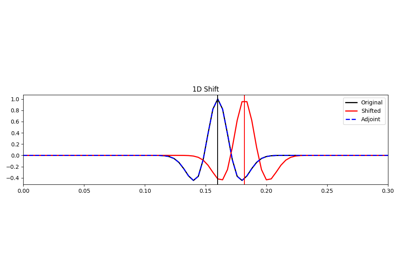# pylops.signalprocessing.Shift¶

pylops.signalprocessing.Shift(dims, shift, dir=0, nfft=None, sampling=1.0, real=False, engine='numpy', dtype='complex128', **kwargs_fftw)[source]

Shift operator

Apply fractional shift in the frequency domain along a specific direction dir of a multi-dimensional array of size dim.

Parameters: dims : tuple Number of samples for each dimension shift : float Fractional shift to apply in the same unit as sampling. dir : int, optional Direction along which FFT is applied. nfft : int, optional Number of samples in Fourier Transform (same as input if nfft=None) sampling : float, optional Sampling step $$\Delta t$$. real : bool, optional Model to which fft is applied has real numbers (True) or not (False). Used to enforce that the output of adjoint of a real model is real. engine : str, optional Engine used for fft computation (numpy, scipy, or fftw). Choose numpy when working with CuPy arrays. dtype : str, optional Type of elements in input array. **kwargs_fftw Arbitrary keyword arguments for pyfftw.FTTW ValueError If dims is provided and dir is bigger than len(dims) NotImplementedError If engine is neither numpy, scipy, nor fftw

Notes

The Shift operator applies the forward Fourier transform, an element-wise complex scaling, and inverse fourier transform

$\mathbf{y}= \mathbf{F}^{-1} \mathbf{S} \mathbf{F} \mathbf{x}$

Here $$\mathbf{S}$$ is a diagonal operator that scales the Fourier transformed input by $$e^{-j2\pi f t_S}$$, where $$t_S$$ is the chosen shift.

Attributes: shape : tuple Operator shape explicit : bool Operator contains a matrix that can be solved explicitly (True) or not (False)

## Examples using pylops.signalprocessing.Shift¶Shift